Courses

# NCERT Solutions(Part - 1) - Algebraic Expressions Class 7 Notes | EduRev

## Class 7 : NCERT Solutions(Part - 1) - Algebraic Expressions Class 7 Notes | EduRev

The document NCERT Solutions(Part - 1) - Algebraic Expressions Class 7 Notes | EduRev is a part of the Class 7 Course Mathematics (Maths) Class 7.
All you need of Class 7 at this link: Class 7

EXERCISE 12.1
Q.1. Get the algebraic expressions in the following cases using variables, constants, and arithmetic operations:
(i) Subtraction of z from y.
Solution. y - z
(ii) One-half of the sum of numbers x and y.
Solution. (x + y)/2
(iii) The number z multiplied by itself.
Solution. Z2
(iv) One-fourth of the product of numbers p and q.
Solution. pq/4
(v) Numbers x and y both squared and added.
Solution. x2 + y2
(vi) Number 5 added to three times the product of m and n.
Solution. 3mn + 5
(vii) A product of numbers y and z subtracted from 10.
Solution. 10 - yz
(viii) Sum of numbers a and b subtracted from their product.
Solution. ab - (a + b)

Q.2. (i) Identify the terms and their factors in the following expressions, show the terms and factors by tree diagram:
(a) x - 3
Solution.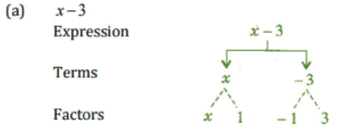(b) 1 + x + x2
Solution.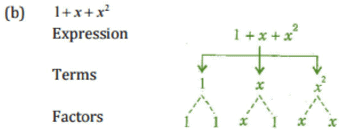(c) y - y3
Solution.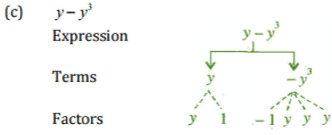(d) 5xy2 + 7x2y
Solution.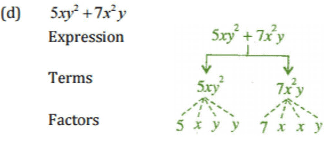(e) -ab + 2b2 - 3a2
Solution.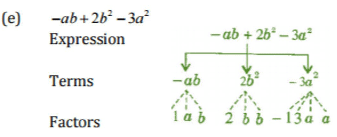(ii) Identify the terms and factors in the expressions given below:

(a) -4x + 5
Solution. (a) -4x+ 5
Terms: -4x,5
Factors: -4,x ; 5

(b) -4x + 5y
Solution. (b) -4x + 5y
Terms: -4x, 5y
Factors: -4,x ; 5,y

(c) 5y + 3y2
Solution. (c) 5y + 3y2
Terms: 5y,3y2
Factors: 5, y ; 3,y,y

(d) xy + 2x2y2
Solution.(d) xy+2x2y2
Terms: xy,2x2y
Factors: x,y ; 2x,x,y,y

(e) pq + q
Solution. (e) pq+q
Terms: pq,q
Factors: p,q ; q

(f) 1.2ab - 2.4b + 3.6a
Solution.(f) 1.2ab-2.4b+3.6a
Terms: 1,2ab.-2.4b,3 6a
Factors: 1.2.a.b ; -2.4,6 ; 3.6,a

(g)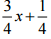Solution.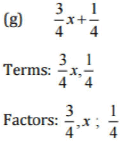(h) 0.1 p2 + 0.2q2
Solution. (h) 0.1p2+0.2q2
Terms: 0.1 p2,0.2q2
Factors: 0. 1,p,p, ; 0.2, q,q

Q.3. Identify the numerical coefficients of terms (other than constants) in the following expressions:
(i) 5 - 3t2
(ii) 1 + t + t2 + t2
(iii) x + 2xy + 3y
(iv) 100m + 1000n
(v) -p2q2 + 7pq
(vi) 1.2a + 0.8b
(vii) 3.14 r2
(viii) 2(l+b)
(ix) 0.1y + 0.01y
2
Solution.

 S.No. Expression Terms Numerical Coefficient (i) 5-3t2 -3t1 -3 (ii) 1+t+t2+t3 t 1 t2 1 t3 1 (iii) x + 2xy + 3y x 1 2xy 2 3y 3 (iv) 100m+1000n 100 m 100 1000 n 1000 (v) -p2q2+7 pq -p2q2 -1 7 pq 7 (vi) 1.2a+0.8b 1.2 a 1.2 0.8b 0.8 (vii) 3.14 r2 3.14 r2 3.14 (viii) 2 (l + b) = 2l+ 2b 2l 2 2b 2 (ix) 0.1y + 0.01y2 0.1y 0.1 0.01y2 0.01

Q.4.

(a) Identify terms which contain x and give the coefficient of x.
(i) y2x + y
(ii) 13y2 - 8yx
(iii) x + y + 2
(iv) 5 + z + zx
(v) 1 + x + xy
(vi) 12xy2 + x25
(vii) 7x + xy2

(b) Identify terms which contain y2 and give the coefficient of y2.
(i) 8 - xy2
(ii) 5y2 + 7x
(iii) 2x2y - 15xy2 + 7y
2
Solution.
(a)

 S.No. Expression Term with factor x Coefficient of x (i) y2x + y y2 x y (ii) 13y2 -8yx -8 yx -8 y (iii) x + y + 2 x 1 (iv) 5 + z + zx zx z (v) 1 + x + xy x 1 xy y (vi) 12xy2 +25 12 xy2 12y2 (vii) 7x+xy2 xy2 y2 7x 7

(b)

 S.No. Expression Term containing y2 Coefficient of y2 (i) 8-xy2 -xy2 -x (ii) 5/+7x 5y2 5 (iii) 2x2y-15xy2 + 7y2 -15xy2 -15x 7y2 7

Q.5. Classify into monomials, binomials and trinomials:
(i) 4y - 7x
(ii) y2
(iii) x + y - xy
(iv) 100
(v) ab - a - b
(vi) 5 - 3t
(vii) 4p2q - 4pq2
(viii) 7mn
(ix) z2 - 3z + 8
(x) a2 + b2
(xi) z2 + z

(xii) 1 + x + x2
Solution.

 S.No. Expression Type of Polynomial (i) 4y-7z Binomial (ii) y2 Monomial (iii) x+y-xy Trinomial (iv) 100 Monomial (V) ab-a-b Trinomial (Vi) 5-3t Binomial (vii) 4p2q-4pq2 Binomial (viii) 7mn Monomial (ix) z2-3z + 8 Trinomial (X) a2 + b2 Binomial (xi) z2 +z Binomial (xii) 1 + x + x2 Trinomial

Q.6. State whether a given pair of terms is of like or unlike terms:

(i) 1,100
(ii)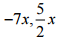(iii) -29x, -29y
(iv) 14xy, 42 yx
(v) 4m2p, 4mp2
(vi) 12xz, 12x2 z2

Solution.

 S.No. Pair of terms Like / Unlike terms (i) 1, 100 Like terms (ii)Like terms (iii) -29x,-29y Unlike terms (iv) 14xy,42yx Like terms (v) 4m2p,4mp2 Unlike terms (vi) 12xz,12x2z2 Unlike terms

Q.7. Identify like terms in the following:
(a) -xy2, -4yx2, 8x2, 2xy2, 7y,  -11x2  - 100x, - 11yx, 20x2y, -6x2, y, 2xy, 3x
Solution.
(i) -xy2,2 xy2
(ii) -4yx2 , 20x2y
(iii) 8x2,-11x2,-6x2
(iv) 7y, y
(v) -100x, 3x
(vi) -11yx, 2xy

(b) 10pq, 7p, 8q, -p2q2, -7qp, -100q, -23, 12q2p2, -5p2, 41,2405 p, 78qp, 13p2q, qp2, 701p2
Solution.
(i) 10 pq - 7 pq,78 pq
(ii) 7p, 2405 p
(iii) 8q,- 100q
(iv) -p2q2, 12p2q2
(v) -12,41
(vi)
-5p2,701p2
(vii) 13 p2q,qp2

EXERCISE 12.2
Q.1. Simplify combining like terms:
(i) 21b – 32 + 7b – 20b
Solution:

When term have the same algebraic factors, they are like terms.
Then,
= (21b + 7b – 20b) – 32
= b (21 + 7 – 20) – 32
= b (28 - 20) – 32
= b (8) - 32
= 8b - 32
(ii) – z2 + 13z2 – 5z + 7z3 – 15z
Solution:
When term have the same algebraic factors, they are like terms.
Then,
= 7z3 + (-z2 + 13z2) + (-5z – 15z)
= 7z3 + z2 (-1 + 13) + z (-5 - 15)
= 7z3 + z2 (12) + z (-20) + 7z3
= 7z3 + 12z2 – 20z + 7z3

(iii) p – (p – q) – q – (q – p)
Solution:
When term have the same algebraic factors, they are like terms.
Then,
= p – p + q – q – q + p
= p – q

(iv) 3a – 2b – ab – (a – b + ab) + 3ab + b – a
Solution:
When term have the same algebraic factors, they are like terms.
Then,
= 3a – 2b – ab – a + b – ab + 3ab + b – a
= 3a – a – a – 2b + b + b – ab – ab + 3ab
= a (1 – 1- 1) + b (-2 + 1 + 1) + ab (-1 -1 + 3)
= a (1 - 2) + b (-2 + 2) + ab (-2 + 3)
= a (1) + b (0) + ab (1)
= a + ab

(v) 5x2y – 5x2 + 3yx2 – 3y2 + x2 – y2 + 8xy2 – 3y2
Solution:
When term have the same algebraic factors, they are like terms.
Then,
= 5x2y + 3yx2 – 5x2 + x2 – 3y2 – y2 – 3y2
= x2y (5 + 3) + x2 (- 5 + 1) + y2 (-3 – 1 -3) + 8xy2
= x2y (8) + x2 (-4) + y2 (-7) + 8xy2
= 8x2y - 4x2 – 7y2 + 8xy2

(vi) (3y2 + 5y – 4) – (8y – y2 – 4)
Solution:
When term have the same algebraic factors, they are like terms.
Then,
= 3y2 + 5y – 4 – 8y + y2 + 4
= 3y2 + y2 + 5y – 8y – 4 + 4
= y2 (3 + 1) + y (5 - 8) + (-4 + 4)
= y2 (4) + y (-3) + (0)
= 4y2 – 3y

(i) 3mn, – 5mn, 8mn, – 4mn
Solution: When term have the same algebraic factors, they are like terms.
Then, we have to add the like terms
= 3mn + (-5mn) + 8mn + (- 4mn)
= 3mn – 5mn + 8mn – 4mn
= mn (3 – 5 + 8 - 4)
= mn (11 - 9)
= mn (2)
= 2mn

(ii) t – 8tz, 3tz – z, z – t
Solution: When term have the same algebraic factors, they are like terms.
Then, we have to add the like terms
= t – 8tz + (3tz - z) + (z - t)
= t – 8tz + 3tz – z + z - t
= t – t – 8tz + 3tz – z + z
= t (1 - 1) + tz (- 8 + 3) + z (-1 + 1)
= t (0) + tz (- 5) + z (0)
= - 5tz

(iii) – 7mn + 5, 12mn + 2, 9mn – 8, – 2mn – 3
Solution: When term have the same algebraic factors, they are like terms.
Then, we have to add the like terms
= - 7mn + 5 + 12mn + 2 + (9mn - 8) + (- 2mn - 3)
= - 7mn + 5 + 12mn + 2 + 9mn – 8 - 2mn - 3
= - 7mn + 12mn + 9mn – 2mn + 5 + 2 – 8 – 3
= mn (-7 + 12 + 9 - 2) + (5 + 2 – 8 - 3)
= mn (- 9 + 21) + (7 - 11)
= mn (12) – 4
= 12mn - 4

(iv) a + b – 3, b – a + 3, a – b + 3
Solution: When term have the same algebraic factors, they are like terms.
Then, we have to add the like terms
= a + b – 3 + (b – a + 3) + (a – b + 3)
= a + b – 3 + b – a + 3 + a – b + 3
= a – a + a + b + b – b – 3 + 3 + 3
= a (1 – 1 + 1) + b (1 + 1 - 1) + (-3 + 3 + 3)
= a (2 -1) + b (2 -1) + (-3 + 6)
= a (1) + b (1) + (3)
= a + b + 3

(v) 14x + 10y – 12xy – 13, 18 – 7x – 10y + 8xy, 4xy
Solution:
When term have the same algebraic factors, they are like terms.
Then, we have to add the like terms
= 14x + 10y – 12xy – 13 + (18 – 7x – 10y + 8xy) + 4xy
= 14x + 10y – 12xy – 13 + 18 – 7x – 10y + 8xy + 4xy
= 14x – 7x + 10y– 10y – 12xy + 8xy + 4xy – 13 + 18
= x (14 - 7) + y (10 - 10) + xy(-12 + 8 + 4) + (-13 + 18)
= x (7) + y (0) + xy(0) + (5)
= 7x + 5

(vi) 5m – 7n, 3n – 4m + 2, 2m – 3mn – 5
Solution:
When term have the same algebraic factors, they are like terms.
Then, we have to add the like terms
= 5m – 7n + (3n – 4m + 2) + (2m – 3mn - 5)
= 5m – 7n + 3n – 4m + 2 + 2m – 3mn – 5
= 5m – 4m + 2m – 7n + 3n – 3mn + 2 – 5
= m (5 - 4 + 2) + n (-7 + 3) – 3mn + (2 - 5)
= m (3) + n (-4) – 3mn + (-3)
= 3m – 4n – 3mn - 3

(vii) 4x2y, – 3xy2, –5xy2, 5x2y
Solution:

When term have the same algebraic factors, they are like terms.
Then, we have to add the like terms
= 4x2y + (-3xy2) + (-5xy2) + 5x2y
= 4x2y + 5x2y – 3xy2 – 5xy2
= x2y (4 + 5) + xy2 (-3 - 5)
= x2y (9) + xy2 (- 8)
= 9x2y – 8xy2

(viii) 3p2q2 – 4pq + 5, – 10 p2q2, 15 + 9pq + 7p2q2
Solution: When term have the same algebraic factors, they are like terms.
Then, we have to add the like terms
= 3p2q2 – 4pq + 5 + (- 10p2q2) + 15 + 9pq + 7p2q2
= 3p2q2 – 10p2q2 + 7p2q2 – 4pq + 9pq + 5 + 15
= p2q2 (3 -10 + 7) + pq (-4 + 9) + (5 + 15)
= p2q2 (0) + pq (5) + 20
= 5pq + 20

(ix) ab – 4a, 4b – ab, 4a – 4b
Solution:
When term have the same algebraic factors, they are like terms.
Then, we have to add the like terms
= ab – 4a + (4b – ab) + (4a – 4b)
= ab – 4a + 4b – ab + 4a – 4b
= ab – ab – 4a + 4a + 4b – 4b
= ab (1 -1) + a (4 - 4) + b (4 - 4)
= ab (0) + a (0) + b (0)
= 0

(x) x2 – y2 – 1, y2 – 1 – x2, 1 – x2 – y2
Solution:
When term have the same algebraic factors, they are like terms.
Then, we have to add the like terms
= x2 – y2 – 1 + (y2 – 1 – x2) + (1 – x2 – y2)
= x2 – y2 – 1 + y2 – 1 – x2 + 1 – x2 – y2
= x2 – x2 – x2 – y2 + y2 – y2 – 1 – 1 + 1
= x2 (1 – 1- 1) + y2 (-1 + 1 - 1) + (-1 -1 + 1)
= x2 (1 - 2) + y2 (-2 +1) + (-2 + 1)
= x2 (-1) + y2 (-1) + (-1)
= -x2 – y2 -1

Q.3. Subtract:
(i) -5y2 from y2
Solution.
y2 - (-5y2) = y2 + 5y2 = 6y2
(ii) 6xy from -12xy

Solution.
-12xy -(6xy) = -12xy - 6xy = -18xy
(iii) (a - b) from (a + b)

Solution.
(a + b)-(a -b) = a + b -a + b
= a - a + b + b = 2b
(iv) a (b - 5) from b (5 - a)

Solution.
= b (5 - a)-a (b -5)
= 5b - ab - ab + 5a
= 5b - 2ab+5a
= 5a + 5b -2ab
(v) -m2 + 5mn from 4m2 - 3mn + 8

Solution.
= 4m2 - 3mn + 8 - (- m2 + 5mn)
= 4m2 - 3mn + 8 + m2 - 5mn
= 4m2 + m2 - 3mn - 5mn + 8
= 5m2 - 8mn + 8
(vi) -x2 +10x - 5 from 5x-10

Solution.
= 5x – 10 – (-x2 + 10x - 5)
= 5x – 10 + x2 – 10x + 5
= x2 + 5x – 10x – 10 + 5
= x2 – 5x - 5

(vii) 5a2 - 7ab + 5b2 from 3ah - 2a2 - 2b2
Solution.
= 3ab – 2a2 – 2b2 – (5a2 – 7ab + 5b2)
= 3ab – 2a2 – 2b2 – 5a2 + 7ab – 5b2
= 3ab + 7ab – 2a2 – 5a2 – 2b2 – 5b2
= 10ab – 7a2 – 7b2

(viii) 4pq - 5q2 - 3p2 from 5p2 + 3q2 - pq
Solution.
= 5p2 + 3q2 – pq – (4pq – 5q2 – 3p2)
= 5p2 + 3q2 – pq – 4pq + 5q2 + 3p2
= 5p2 + 3p2 + 3q2 + 5q2 – pq – 4pq
= 8p2 + 8q2 – 5pq

Q.4. (a) What should be added to x2 +xy+y2 to obtain 2x2 +3xy ?
Solution. (a) Let p should be added.
Then according to question,
x2 + xy + y2 + p = 2x2 + 3xy
⇒ p = 2x2 + 3xy - (x2 + xy + y2)
⇒ p = 2x2 + 3xy - x2 - xy - y2
⇒ p = 2x2 - x2- y2 +3xy - xy
⇒ p = x2 - y2 + 2xy
Hence, x2 - y2 + 2xy should be added.

(b) What should be subtracted from 2a + 8b+10 to get -3a + 7b + 16?
Solution. (b) Let q should be subtracted.
Then according to question, 2a + 8b + 10-q = -3a + 7b + 16
⇒ -q = -3a +7b + 16 - (2a + 8b + 10)
⇒ -q = -3a + 7b + 16 - 2a - 8b - 10
⇒ - q = -3a - 2a + 7b - 8b + 16 - 10
⇒ -q = -5a - b + 6
⇒ q = - (- 5a - b + -6)
⇒ q = 5a + b - 6

Q.5. What should be taken away from 3x2- 4y2 + 5xy + 20 to obtain - x2 - y2 + 6xy + 20 ?
Solution. Let q should be subtracted.
Then according to question,
3x- 4y2 + 5xy + 20 -q = -x2 - y2 + 6xy + 20
⇒ q = 3x2 - 4y2 + 5xy + 20 - (-x2 - y+ 6xy + 20)
⇒ q = 3x2 - 4y2 + 5xy+ 20 + x2 + y2 - 6xy - 20
⇒ q = 3x2 + x2 - 4y+y+ 5xy - 6xy + 20-20
⇒ q =  4x2 - 3y2 -  xy + 0
Hence, 4x2 -3y2 -xy should be subtracted.

Q.6. (a) From the sum of 3x - y + 11 and - y - 11, subtract 3x - y - 11.
Solution. First we have to find out the sum of 3x – y + 11 and – y – 11
= 3x – y + 11 + (-y - 11)
= 3x – y + 11 – y – 11
= 3x – y – y + 11 – 11
= 3x – 2y
Now, subtract 3x – y – 11 from 3x – 2y
= 3x – 2y – (3x – y - 11)
= 3x – 2y – 3x + y + 11
= 3x – 3x – 2y + y + 11
= -y + 11

(b) From the sum of 4 + 3x and 5 - 4x + 2x2, subtract the sum of 3x2 - 5x and -x+ 2x + 5.
Solution. First we have to find out the sum of 4 + 3x and 5 – 4x + 2x2
= 4 + 3x + (5 – 4x + 2x2)
= 4 + 3x + 5 – 4x + 2x2
= 4 + 5 + 3x – 4x + 2x2
= 9 - x + 2x2
= 2x2 – x + 9     … [equation 1]
Then, we have to find out the sum of 3x2 – 5x and – x2 + 2x + 5

Offer running on EduRev: Apply code STAYHOME200 to get INR 200 off on our premium plan EduRev Infinity!

## Mathematics (Maths) Class 7

165 videos|222 docs|45 tests

,

,

,

,

,

,

,

,

,

,

,

,

,

,

,

,

,

,

,

,

,

;